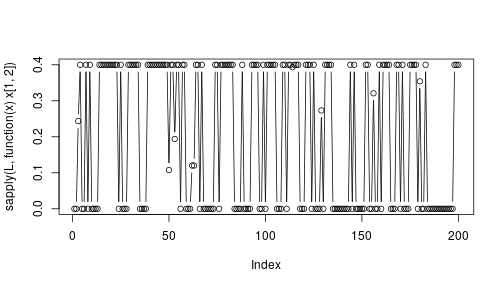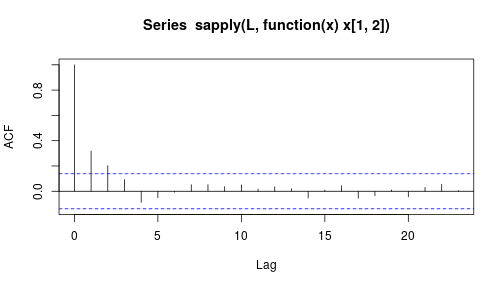An example with 3 banks

This document describe a toy example for the use of the package systemicrisk.

library(systemicrisk)

Suppose we are dealing with 3 banks and that they have total interbank liabilities/assets given by the following:

l <- c(1,2.5,3)
a <- c(0.7,2.7,3.1)

Suppose we are assuming $$p=0.5$$ and $$\lambda=0.25$$. Then we can run the Gibbs sampler to get a sample of the liabilities matrix condtional on $$l$$ and $$a$$.

L <- sample_ERE(l,a,p=0.5,lambda=0.25,nsamples=200,thin=20,burnin=10)

Some examples of the matrics generated are below.

L[]
##      [,1] [,2] [,3]
## [1,]  0.0  0.0  1.0
## [2,]  0.4  0.0  2.1
## [3,]  0.3  2.7  0.0
L[]
##      [,1] [,2] [,3]
## [1,]  0.0  0.0  1.0
## [2,]  0.4  0.0  2.1
## [3,]  0.3  2.7  0.0
L[]
##           [,1]      [,2]      [,3]
## [1,] 0.0000000 0.2436671 0.7563329
## [2,] 0.1563329 0.0000000 2.3436671
## [3,] 0.5436671 2.4563329 0.0000000

Diagnostic of the R-output

All the caveats of MCMC algorithms apply. In particular it is useful to plot the paths of the individual liabilities, which we do below for the liabilities of Bank 1 towards Bank 2.

plot(sapply(L,function(x)x[1,2]),type="b")Also, the autocorrelation function should decline quickly.

acf(sapply(L,function(x)x[1,2]))In this case it decays quickly below the white-noise threshold (the horizontal dashed lines).

Default of banks

To be able to talk about default of banks we need to know the external assets and liabilities of the banks. Suppose we assume the following:

ea <- c(1,1,1)
el <- c(1,1,1)
default(L[],ea=ea,el=el)$defaultind ##  1 0 1 default(L[],ea=ea,el=el)$defaultind
##  1 0 1
default(L[],ea=ea,el=el)$defaultind ##  1 0 1 Below we apply two different default algorithms (without default costs and with default costs) to the liabilities matrices and compute the average number of times each bank defaults. rowMeans(sapply(L, function(Lakt) default(Lakt,ea=ea,el=el)$defaultind))
##  1.000 0.000 0.515
rowMeans(sapply(L, function(Lakt) default(Lakt,ea=ea,el=el,alpha=0.98,beta=0.98)\$defaultind))
##  1 0 1